# SATs Mathematics Reasoning (Paper 3) in the Style of the Key Stage 2 National Tests

In this assessment, students will be able to complete a timed Reasoning paper in the style of Key Stage 2 SATs.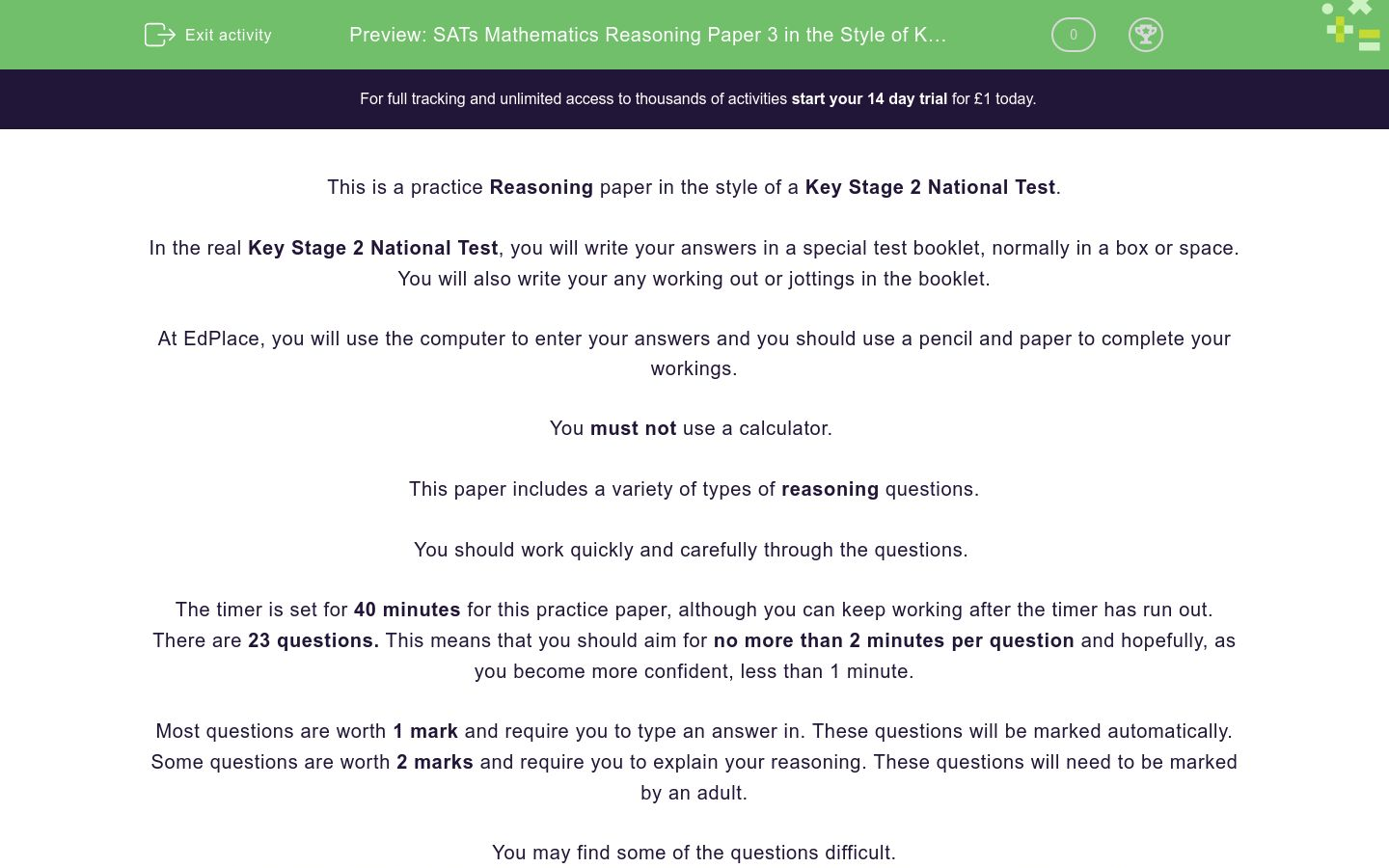Key stage:  KS 2

Curriculum topic:   SATs Practice Papers

Curriculum subtopic:   Reasoning Practice Papers

Difficulty level:### QUESTION 1 of 10

This is a practice Reasoning paper in the style of a Key Stage 2 National Test.

In the real Key Stage 2 National Test, you will write your answers in a special test booklet, normally in a box or space.

You will also write your any working out or jottings in the booklet.

At EdPlace, you will use the computer to enter your answers and you should use a pencil and paper to complete your workings.

You must not use a calculator.

This paper includes a variety of types of reasoning questions.

You should work quickly and carefully through the questions.

The timer is set for 40 minutes for this practice paper, although you can keep working after the timer has run out.

There are 23 questions. This means that you should aim for no more than 2 minutes per question and hopefully, as you become more confident, less than 1 minute.

Most questions are worth 1 mark and require you to type an answer in. These questions will be marked automatically.

Some questions are worth 2 marks and require you to explain your reasoning. These questions will need to be marked by an adult.

You may find some of the questions difficult.

If you are struggling to answer a question do not waste time on it, but move onto the next question.

Disclaimer:

We have no affiliation to the Standards and Testing Agency (STA) and these questions represent our own unique content developed by EdPlace Key Stage 2 Maths authors.

None of the content displayed here has been supplied by the STA or any other third party suppliers.

Select the two decimals which are closest in value to each other.

0.3      0.03      0.33      0.1      0.01

0.3

0.03

0.33

0.1

0.01

Find the value of b in this equation.

5 + 5b = 105

Bill wants to fill a saucepan with water.

The saucepan holds 2 litres.

A jug holds 500 millilitres.

How many jugs of water are needed to fill the saucepan?

Write the missing number in the calculation below:

? - 1209 = 357

A group of 8 children and 3 adults go to the cinema.

Children cost £3.95 each.

How much does it cost altogether?

Show your answer as a decimal with pence after the decimal point.

Place each number into the correct section, marked A, B, C or D.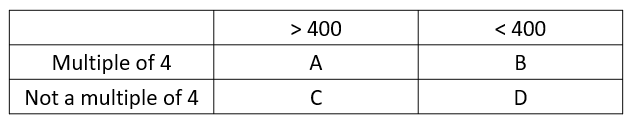## Column B

A
414
B
360
C
528
D
234

Jack has 49p.

Jill has 57p.

They share what they have equally between them.

How much does each of them get in pence?

The pictogram shows the number of comics and books read, and the number of films watched by four children in a year.

Who read the same number of books as the number of comics read by Nikita?Jack

Nikita

Aisha

Dan

Which of the following shapes are not quadrilaterals?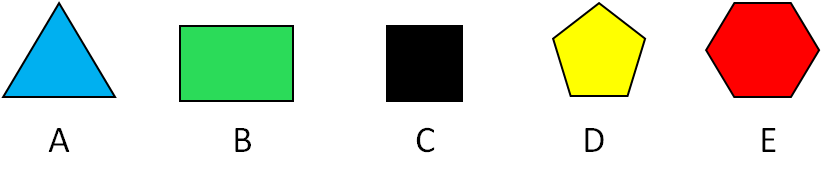A

B

C

D

E

The diagram below shows an isosceles triangle inside a rectangle.Calculate the value of angle x in degrees.

A

B

C

D

E

Ben went to sleep at midnight.

He spends seven minutes reading his mystery book, five minutes putting his pyjamas on and three minutes cleaning his teeth.

What time is it when Ben starts his bedtime routine?

Find two answers which are correct.

Twenty to twelve.

11:45 pm

11:40 am

Quarter to 12.

11:55 pm

Place the following lengths in order of size, starting with the smallest.

## Column B

1 (smallest)
5 metres
2
5 miles
3
5 inches
4
5 kilometres
5 (largest)
5 centimetres

The temperatures in Rome, London and Paris on one particular summer's afternoon are shown below.

How many degrees hotter is it in the hottest city compared to the coldest?Find the value of p in this equation.

5(2 + p) = 17.5

Tickets for the theatre cost £15 each.

How much do 27 tickets cost in pounds?

The diagram shows two similar rectangles.Calculate the area of rectangle B in cm².

Here is a parallelogram drawn on a graph.

It is translated as follows: 3R 2U

What are the coordinates of the point to which the point (4, 3) will go?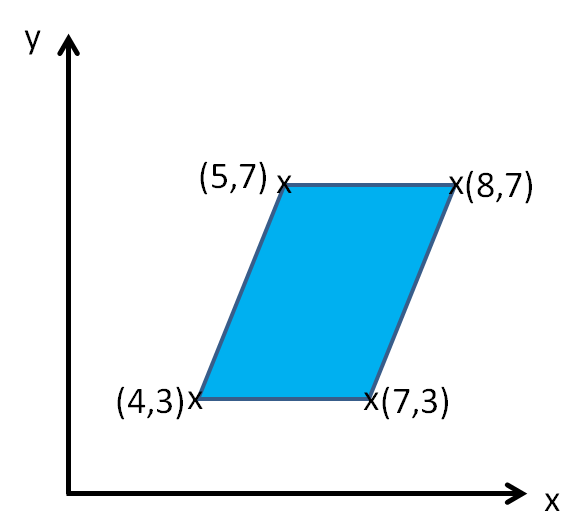(5, 7)

(7, 5)

(7, 3)

(4, 5)

(6, 6)

(8, 5)

A rectangle is shown below with a line touching one corner.

Calculate the size of angle x in degrees.Do not use a protractor or angle measurer.

This pie chart shows the results of a survey on favourite crisp flavours.

160 people took part in the survey.How many chose Salt and Vinegar or Ready Salted?

How do you know? Show your working.

Envelopes are sold in small packs of 15 and large packs of 22.

Sid needs 72 envelopes.

How many of each size pack should Sid buy so as to have as little waste as possible?

Can you explain why?

The points A and B are shown on the coordinate axes below.

A straight, line is drawn through point M and meets the x-axis at 90°.

For one mark, what is the position of the point M?

For a second mark, name 4 possible points which lay on the line drawn.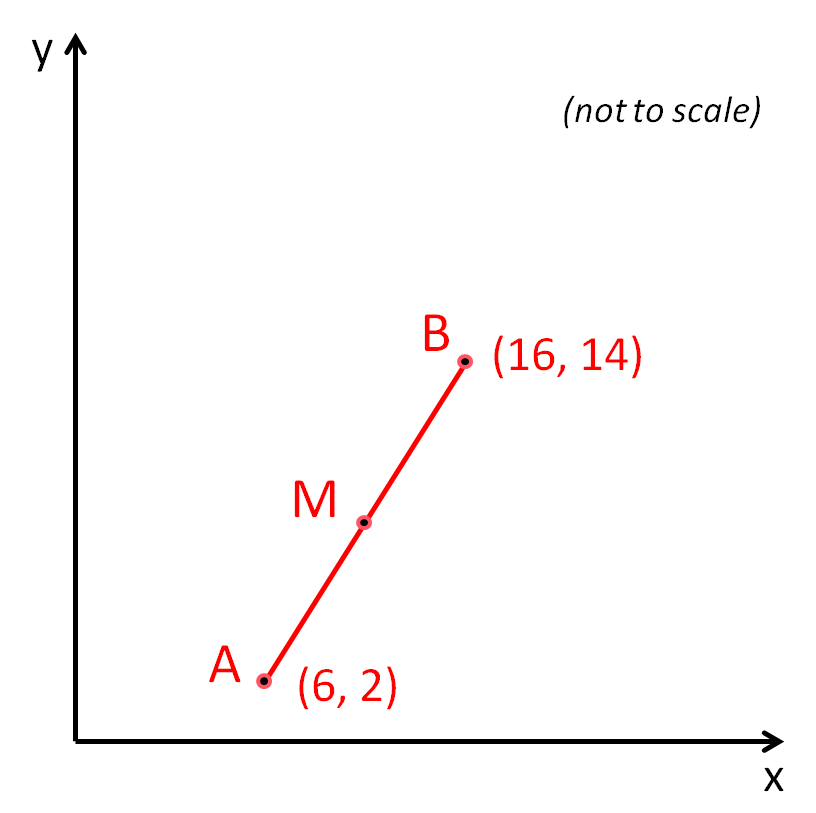Here is a 3 cm by 4 cm rectangle.The following shape is made of four of these 3 cm by 4 cm rectangles.Olivia correctly calculates that the first rectangle has a perimeter of 12 cm.

She then states that the perimeter of the larger shape must be 48 cm.

Is Olivia correct? Explain why.

The day temperature and the night temperature in Jack's garden are shown below.

What is the difference between the two readings?• Question 1

Select the two decimals which are closest in value to each other.

0.3      0.03      0.33      0.1      0.01

0.03
0.01
EDDIE SAYS
The two correct decimals are 0.03 and 0.01. This is because 0.3 and 0.33 are 0.03 apart and 0.01 and 0.03 are only 0.02 apart.
• Question 2

Find the value of b in this equation.

5 + 5b = 105

20
EDDIE SAYS
b represents 20. We can solve this problem by using inverses. 5 + 5b = 105 First, subtract 5 from both sides of the equation. 5b = 100 5b means 5 lots of b. 100 ÷ 5 = 20
• Question 3

Bill wants to fill a saucepan with water.

The saucepan holds 2 litres.

A jug holds 500 millilitres.

How many jugs of water are needed to fill the saucepan?

4
EDDIE SAYS
The answer is 4. Remember: 2 litres = 2000 millilitres. Divide the volume of water held by the pan, by the volume held by the jug. 2000 ÷ 500 = 4 Therefore, four jugs are needed to fill the saucepan.
• Question 4

Write the missing number in the calculation below:

? - 1209 = 357

EDDIE SAYS
The missing number is 1566. To find the missing number, add together the other numbers. Work out 357 + 1209 = 1566 Then check: 1566 - 1209 = 357.
• Question 5

A group of 8 children and 3 adults go to the cinema.

Children cost £3.95 each.

How much does it cost altogether?

Show your answer as a decimal with pence after the decimal point.

52.45
EDDIE SAYS
The total cost is £52.45. Firstly, work out the cost of the adults' tickets. You could use written multiplication: 3 × 6.95 = 20.85 Or you could use this mental method: (3 × 7) - (3 × 0.05) = 21 - 0.15 = 20.85 Then work out the cost of the children's tickets: 8 × 3.95 = 31.60 or (8 × 4) - (8 × 0.05) = 32 - 0.40 = 31.60 Then add the costs together. 20.85 + 31.60 = 52.45
• Question 6

Place each number into the correct section, marked A, B, C or D.## Column B

A
528
B
360
C
414
D
234
EDDIE SAYS
528 belongs in A. 360 belongs in B. 414 belongs in C. 234 belongs in D. You could do the divisions or.... remember that:
A number is divisible by 4 if the number formed by its last two digits is divisible by 4.

528 is divisible by 4 because 28 is divisible by 4.
360 is divisible by 4 because 60 is divisible by 4.
414 is not divisible by 4 because 14 is not divisible by 4.
234 is not divisible by 4 because 34 is not divisible by 4.
• Question 7

Jack has 49p.

Jill has 57p.

They share what they have equally between them.

How much does each of them get in pence?

53
EDDIE SAYS
They each get 57 pence. First, add together the amounts to get £1.06 (or 106 pence). 49 + 57 = 106 Next, divide this in half mentally. 106 ÷ 2 = 53
• Question 8

The pictogram shows the number of comics and books read, and the number of films watched by four children in a year.

Who read the same number of books as the number of comics read by Nikita?Aisha
EDDIE SAYS
The answer is Aisha. Each comic book symbol stands for six comics. Nikita read 24 comics. 4 × 6 = 24 Each book symbol stands for 4 books. Aisha read 24 books. 6 × 4 = 24
• Question 9

Which of the following shapes are not quadrilaterals?A
D
E
EDDIE SAYS
The correct answers are A, D and E. Quadrilaterals have exactly four sides. Did you spot the quadrilaterals? B and C are quadrilaterals. A, D and E are not quadrilaterals.
• Question 10

The diagram below shows an isosceles triangle inside a rectangle.Calculate the value of angle x in degrees.

EDDIE SAYS
The answer is 40°. The obtuse angle in the large isosceles triangle must be 100° as angles on a straight line add up to 180° and the two small isosceles triangles therefore, have angles of 40°. 180 - 40 - 40 = 100 Isosceles triangles have two equal angles and angles in a triangle also add up to 180°. Therefore, angle x must be 40°. 180 - 100 = 80 80 ÷ 2 = 40
• Question 11

Ben went to sleep at midnight.

He spends seven minutes reading his mystery book, five minutes putting his pyjamas on and three minutes cleaning his teeth.

What time is it when Ben starts his bedtime routine?

Find two answers which are correct.

11:45 pm
Quarter to 12.
EDDIE SAYS
The two correct answers are twenty to twelve and 11:40 pm. Ben spends 15 minutes on his bedtime routine. 7 + 5 + 3 = 12 The time 15 minutes before midnight is 11:40 pm or twenty to twelve.
• Question 12

Place the following lengths in order of size, starting with the smallest.

## Column B

1 (smallest)
5 centimetres
2
5 inches
3
5 metres
4
5 kilometres
5 (largest)
5 miles
EDDIE SAYS
The correct order is 5 cm, 5 inches, 5 metres, 5 km and 5 miles. Two useful facts to remember are: 1 inch ≅ 2.54 cm 5 miles ≅ 8 km
• Question 13

The temperatures in Rome, London and Paris on one particular summer's afternoon are shown below.

How many degrees hotter is it in the hottest city compared to the coldest?17
EDDIE SAYS
The answer is 17 °. The temperature in Rome is 33°, London is 16° and Paris is 24°. So, Rome is the hottest and London is the coldest. The difference between these temperatures is 17 °. 33 - 16 = 17
• Question 14

Find the value of p in this equation.

5(2 + p) = 17.5

1.5
EDDIE SAYS
The answer is 1.5. 5(2 + p) = 17.5 means that five lots of (2 + p) equals 17.5. To find the value of (2 + p), divide: 17 ÷ 5 = 3.5 To find the value of p, subtract the two. 3.5 - 2 = 1.5
• Question 15

Tickets for the theatre cost £15 each.

How much do 27 tickets cost in pounds?

405
EDDIE SAYS
The answer is £405. To find the answer we must multiply. 15 × 27 = 405 Use long multiplication or mental methods to solve this one! For example, 10 tickets will cost £270 and 5 tickets will cost £135. 270 + 135 = 405
• Question 16

The diagram shows two similar rectangles.Calculate the area of rectangle B in cm².

45
EDDIE SAYS
The answer is 45 cm². To find the missing side of rectangle B, we use scale factors. We can see from the sides marked 4 cm and 6 cm, that a scale factor of 1.5 has been used. 4 × 1.5 = 6 So, the missing side must be 7.5 cm. 5 × 1.5 = 7.5 To find the area, use the formula Width × Length. 6 × 7.5 = 45
• Question 17

Here is a parallelogram drawn on a graph.

It is translated as follows: 3R 2U

What are the coordinates of the point to which the point (4, 3) will go?(7, 5)
EDDIE SAYS
The answer is (7,5). A translation is where a shape is moved along and /or up and down. R stands for a movement along to the right. U stands for a movement up. Our shape needs to move three spaces right and two spaces up.
• Question 18

A rectangle is shown below with a line touching one corner.

Calculate the size of angle x in degrees.Do not use a protractor or angle measurer.

55
EDDIE SAYS
The answer is 55 °. Angles on a straight line always equal 180°. Right angles (90°) are always marked with a square angle marker. x = 180 - 35 - 90 = 55
• Question 19

This pie chart shows the results of a survey on favourite crisp flavours.

160 people took part in the survey.How many chose Salt and Vinegar or Ready Salted?

How do you know? Show your working.

EDDIE SAYS
The answer is 60 people. 90° is 1/4 of the circle, so 45° is 1/8 of the circle. 160 ÷ 8 = 20 This is the number of votes for Salt and Vinegar. 160 ÷ 4 = 40 This is the number of votes for Ready Salted. 20 + 40 = 60 This is the total number votes for Salt and Vinegar and Ready Salted.
• Question 20

Envelopes are sold in small packs of 15 and large packs of 22.

Sid needs 72 envelopes.

How many of each size pack should Sid buy so as to have as little waste as possible?

Can you explain why?

EDDIE SAYS
Sid should buy two large and two small packs. 22 + 22 + 15 + 15 = 74 This will leave only 2 spare envelopes. All other combinations mean Sid has more envelopes left over.
• Question 21

The points A and B are shown on the coordinate axes below.

A straight, line is drawn through point M and meets the x-axis at 90°.

For one mark, what is the position of the point M?

For a second mark, name 4 possible points which lay on the line drawn.EDDIE SAYS
Point M is at (11,8). 11 is the midpoint between 6 and 16. 8 is the midpoint between 2 and 14. Points on the line drawn could include any number with an x coordinate of 11. For example (11,0), (11,1), (11,2), (11,3) and so on. Well done if you spotted these, this was a tricky question!
• Question 22

Here is a 3 cm by 4 cm rectangle.The following shape is made of four of these 3 cm by 4 cm rectangles.Olivia correctly calculates that the first rectangle has a perimeter of 12 cm.

She then states that the perimeter of the larger shape must be 48 cm.

Is Olivia correct? Explain why.

EDDIE SAYS
Olivia is not correct. She has multiplied the perimeter of the smaller rectangle by four to get 48 cm. The actual perimeter of the larger shape is 32 cm. Perimeter = top + bottom + left + right = 12 + 12 + 4 + 4 = 32
• Question 23

The day temperature and the night temperature in Jack's garden are shown below.

What is the difference between the two readings?30
EDDIE SAYS
The answer is 30 °. The day temperature is 22 °C and the night temperature is -8 °C. The difference is 22 and -8 = 30 °C.
Try it ---- OR ----

Sign up for a £1 trial so you can track and measure your child's progress on this activity.

### What is EdPlace?

We're your National Curriculum aligned online education content provider helping each child succeed in English, maths and science from year 1 to GCSE. With an EdPlace account you’ll be able to track and measure progress, helping each child achieve their best. We build confidence and attainment by personalising each child’s learning at a level that suits them.

Get started